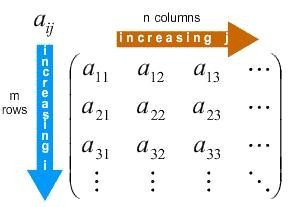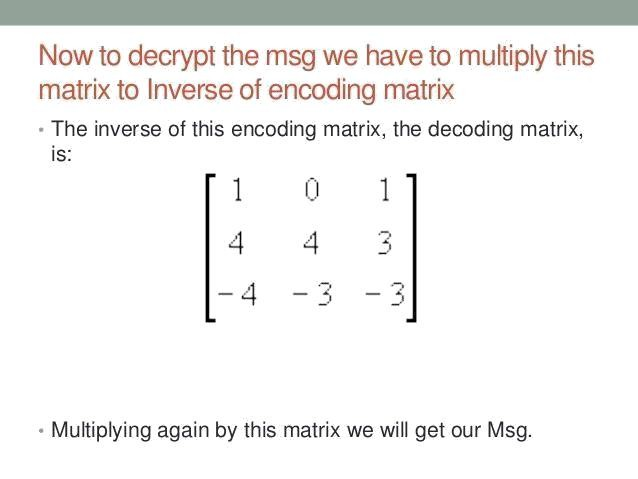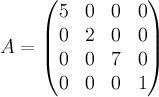×#### Thank you for registering.

One of our academic counsellors will contact you within 1 working day.

Click to Chat

1800-1023-196

+91-120-4616500

CART 0

• 0

MY CART (5)

Use Coupon: CART20 and get 20% off on all online Study Material

ITEM
DETAILS
MRP
DISCOUNT
FINAL PRICE
Total Price: Rs.

There are no items in this cart.
Continue Shopping```Introduction to Matrices

Table of Content

What is a Matrix?

Who coined the term Matrix?

History of Matrix

Uses of Matrix

Structure of Matrix

Usefulness of a Matrix

How to input an entry into a Matrix?

Some terms associated with Matrix

What is a Matrix?

Matrices (singular Matrix) is a cross-formed spreadsheet used to carry out certain mathematical functions. It is a rectangular arrangement of numbers into rows and columns.Who coined the term Matrix?

The first and foremost question that comes to the little analytical minds is that who invented Matrices. The answer is James Sylvester. He was an English mathematician, poet, and also a musician.

History of Matrix

Although, matrices were used by Mayans since ancient times, it was this versatile Englishman who first formulated the true mathematical use of matrices by the year 1850. History of matrices suggest that the origins of mathematical matrices lie with the study of systems of simultaneous linear equations. An important Chinese text from between 300 BC and AD 200, Nine Chapters of the Mathematical Art gives the first known example of the use of matrix methods to solve simultaneous equations. Then James Sylvester, as mentioned above, gave the true mathematical purpose of matrices.Uses of Matrix

Further usage and revolution has brought matrices into the world as we know it today. There are numerous applications of matrices, mostly concentrated to the world of science and mathematics. Since we are interested in its usage in mathematics, matrices find its use in probability theory, statistics, stochastic process, matrix calculus, etc. Matrices are used in economics also to describe systems of economic relationships.

Other usage of the word ‘matrix’ shows that the word has been used by Betrand Russell and Alfred North Whitehead in their book Principia Mathematica in the context of their axiom of reducibility. They proposed this axiom as a means to reduce any function to one of lower type, so that at the bottom the function to its extension.

Structure of Matrix

The picture alongside is an example of a typical normal matrix. Here, the matrix is denoted as A. The terms a11, a12, a13….. a1n, a21, a22,……., a2n, ………., am1, am2……………, amn are termed as the elements of the matrix A. This matrix is divided into m x n elements where m is the entry for each row, and n is the entry for each column. The position of each elements is denoted as aij, where max i = m and max j = n. When the number of rows equals the number of columns, that is, m = n, it is termed as a square matrix.

Usefulness of a Matrix

Uses in Linear Algebra: Matrices come in handy in a lot of ways. One of its important uses is to solve linear equations. By performing certain row operations from the augmented matrix, we can easily find out the values of the variables concerning a set of linear equations. Matrices are also used to solving algorithms in computer programming. Since linear algebra is a very crucial tool in mathematics, matrices have found its vast usage in the world of linear algebra as well.

Scientific Uses: In everyday applications, matrices are used to represent real-world data, such as the traits and habits of a certain population, for example they are also used in geology to measure seismic waves. They are used by the science and research industry to track, record and display the results of a research. In addition to applied science, matrices are also used in the basic sciences. Physicists use matrices to study optics, electrical circuits, and quantum mechanics.

Computer Uses: Computer science also relies heavily on matrices. Tasks such as projecting a three-dimensional image onto a two-dimensional screen and encrypting message codes are two areas in which matrices are used. Matrices and their inverses are necessary to run Internet programs, such as search systems.General Uses: Matrices are also used to segregate data into the correct databases. Before modern advancements, matrix arithmetic helps us calculate the electrical properties of a circuit, with voltage, amperage, resistance, etc. In mathematics, one application of matrix notation supports graph theory. In and adjacency matrix, the integer values of each element indicates how many connections a particular node has.

How to input an entry into a Matrix?

Inputting of an entry in a matrix is done by first entering the row number followed by the column number. It also follows the fact that you can’t directly jump to row number 2 right from the start and then again to row 5 and then row 4 and so on. It follows cardinality, that is, first the entry is inputted in row 1, and then row 2, and so on. Same rule is being followed by the column also.

a11 = This is the first row and the first column = -5

a12 = This is the first row and the second column = 1

a13 = This is the first row and the third column = -3

a21 = This is the second row and the first column = 6

a22 = This is the second row and the second column = 0

a23 = This is the second row and the third column = 2

a31 = This is the third row and the first column = 2

a32= This is the third row and the second column = 6

a33 = This is the third row and the third column = 1This matrix is also an example of square matrix as m = n = 3, that is the number of rows equals the number of columns to 3.

Some terms associated with Matrix

A matrix can be used in simple calculations as well as in complex ones.

The various terms used in matrices are:

Row Vector – A matrix where m = 1 and n = n, that is, the number of row is only one, is termed as row vector.

Column Vector – A matrix where m=m and n=1, that is the number of column is only one, is termed as column vector.

Square Matrix – As explained above, when m=n, that is when the number of rows of a matrix equals the number of columns in a matrix, it is termed as a square matrix.

Diagonal Matrix – A matrix where only the entries are made for m = n, and all the other values of aij is zero. That is for i = j, the entries are made. Generally, a diagonal matrix is a square matrix. The following picture is an example of a diagonal matrix.Transpose of a Matrix – A matrix where the rows and columns of the original gets interchanged. That is if a matrix A has its rows as m and its columns as n, then the transpose of A, denoted as AT, will have its rows as n and its columns as m.

Addition of Matrices – Two matrices can be added only when the number of rows and number of columns of the first matrix equals the number of rows and the number of columns of the second matrix. The addition of matrix is a simple calculation. For every element of aij the first matrix is added to the corresponding element of aij of the second matrix.

Subtraction of Matrices – Two matrices can be subtracted in the similar manner like the addition of matrices.

Multiplication of Matrices – The multiplication of matrices is a complex calculation. It shall be later discussed in the following chapters.

Augmented Matrix – It is a matrix in linear algebra which is obtained by appending the columns of two matrices, say A and B, into a single matrix, usually for the purpose of operations to obtain a result. It is generally calculated by elementary row operations. For a given matrix A and B, the augmented matrix is shown as in the following figure:Invertible Matrix – A square matrix A of order n (m = n = n) is said to be invertible if there exists some n x n matrix B such that AB = BA = I, where AB = Matrix multiplication between A and B, BA = Matrix multiplication between B and A, I is the identity matrix.

Identity Matrix – A square matrix in which all the entries of m and n equals to 1, and the value of the square matrix also equals 1.

Coefficient Matrix – A matrix in linear algebra where all the entries of the matrix is the coefficients of the variables in a set of linear equations.

Size of a Matrix – It is called by the number of rows and columns of a matrix. If there are m rows and n columns, the size of the matrix or the order of the matrix is read as ‘m by n’.

Q1. What is a Matrix?

Sol. As explained above, Matrices (singular Matrix) is a cross-formed spreadsheet used to carry out certain mathematical functions. It is a rectangular arrangement of numbers into rows and columns.

Q2. What is the size of the Matrix?

Sol. It is the number of rows and columns of a matrix. If there are m rows and n columns, the size of the matrix or the order of the matrix is read as ‘m by n’.

Q3. What are the basic operations in a Matrix?

Sol. The basic operations in a matrix are Addition, Subtraction, Multiplication, Transpose of a Matrix, Scalar Multiplication, etc.

Q4. What is the Augmented Matrix?

It is a matrix in linear algebra which is obtained by appending the columns of two matrices, say A and B, into a single matrix, usually for the purpose of operations to obtain a result. It is calculated after carrying out certain elementary row operations.

Watch this Video for more reference

Introduction to Matrices
```### Course Features

• 731 Video Lectures
• Revision Notes
• Previous Year Papers
• Mind Map
• Study Planner
• NCERT Solutions
• Discussion Forum
• Test paper with Video Solution